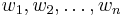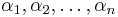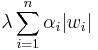# Lasso

## Definition

Lasso, also known as$L^1$-regularization, is a type of regularization where the regularization term is of the following form, where$w_1, w_2, \dots, w_n$ are the unknown parameters of the form being trained for:$\lambda \sum_{i=1}^n | w_i |$

In some cases, due to scaling issues, a lasso may not make direct sense, so we may need additional (predetermined) coefficients$\alpha_1, \alpha_2, \dots, \alpha_n$ to rescale the weights:$\lambda \sum_{i=1}^n \alpha_i |w_i|$

## Effects of lasso

### Summary

Item Value
Convexity The lasso function is convex. Thus, if the original cost function is convex, the regularized cost function is also convex. In particular, it does not destroy the ability to apply optimization methods that rely solely on convexity.
Differentiability The lasso function is differentiable at most points except where one of the weights becomes zero (with partial derivative being undefined in the direction parallel to that weight vector). This can come in the way of iterative application of gradient descent since the gradient vector is undefined. However, setting partial derivative to zero when it is undefined generally works.
Type of model generated Lasso regression pushes towards models where some parameters become precisely zero. It will also generally push those features to be zero that can help distinguish fewer examples, so for instance if a dense feature and a sparser feature both play a similar predictive role, lasso will tend to set the sparser feature to zero. For more, see comparison of lasso and ridge regularization.